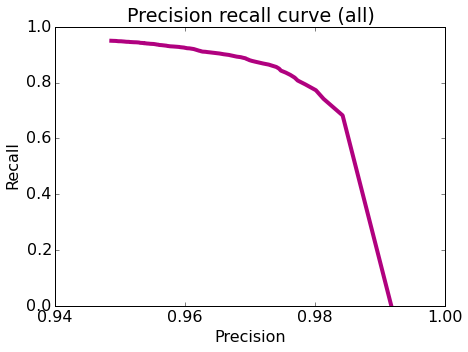# Precision and Recall¶

The goal of this assignment is to understand precision-recall in the context of classifiers.

In :
%%javascript
$.getScript('https://kmahelona.github.io/ipython_notebook_goodies/ipython_notebook_toc.js')  In : import graphlab from __future__ import division import numpy as np import string graphlab.canvas.set_target('ipynb')  In : products = graphlab.SFrame('amazon_baby.gl/')  # Preparations¶ ## Extract word counts and sentiments¶ We compute the word counts for individual words and extract positive and negative sentiments from ratings. In : def remove_punctuation(text): return text.translate(None, string.punctuation) # Remove punctuation, count words review_clean = products['review'].apply(remove_punctuation) products['word_count'] = graphlab.text_analytics.count_words(review_clean) # Drop neutral sentiment reviews, +/-1 to others. products = products[products['rating'] != 3] products['sentiment'] = products['rating'].apply(lambda rating : +1 if rating > 3 else -1)  In : products.head(1)  Out: name review rating word_count sentiment Planetwise Wipe Pouch it came early and was not disappointed. i love ... 5.0 {'and': 3L, 'love': 1L, 'it': 3L, 'highly': 1L, ... 1 [1 rows x 5 columns] ## Training and test split¶ In : train_data, test_data = products.random_split(.8, seed=1)  ## Train a logistic classifier¶ In : model = graphlab.logistic_classifier.create(train_data, target='sentiment', features=['word_count'], validation_set=None, verbose=False)  # Model Evaluation¶ ## Accuracy¶ One performance metric we will use for our more advanced exploration is accuracy. Recall that the accuracy is given by $$\mbox{accuracy} = \frac{\mbox{# correctly classified data points}}{\mbox{# total data points}}$$ In : accuracy= model.evaluate(test_data, metric='accuracy')['accuracy'] print "Test Accuracy: %s" % accuracy  Test Accuracy: 0.914536837053  ## Majority class prediction¶ The majority class classifier is a baseline (i.e reference) model for a point of comparison with a more sophisticated classifier. The majority classifier model predicts the majority class for all data points. Typically, a good model should beat the majority class classifier. Since the majority class in this dataset is the positive class (i.e., there are more positive than negative reviews), the accuracy of the majority class classifier can be computed as follows: In : baseline = len(test_data[test_data['sentiment'] == 1])/len(test_data) print "Baseline accuracy (majority class classifier): %s" % baseline  Baseline accuracy (majority class classifier): 0.842782577394  ## Confusion Matrix¶ The accuracy, while convenient, does not tell the whole story. For a fuller picture, we turn to the confusion matrix. In the case of binary classification, the confusion matrix is a 2-by-2 matrix laying out correct and incorrect predictions made in each label as follows: In : confusion_matrix = model.evaluate(test_data, metric='confusion_matrix')['confusion_matrix'] confusion_matrix  Out: target_label predicted_label count -1 -1 3798 -1 1 1443 1 -1 1406 1 1 26689 [4 rows x 3 columns] ## Computing the cost of mistakes¶ Suppose you know the costs involved in each kind of mistake: \$100 for each false positive and \\$1 for each false negative. What is the cost of the model?

In :
1443*100+1406

Out:
145706

## Precision and Recall¶

You may not have exact dollar amounts for each kind of mistake. Instead, you may simply prefer to reduce the percentage of false positives to be less than, say, 3.5% of all positive predictions. This is where precision comes in:

$$[\text{precision}] = \frac{[\text{# positive data points with positive predicitions}]}{\text{[# all data points with positive predictions]}} = \frac{[\text{# true positives}]}{[\text{# true positives}] + [\text{# false positives}]}$$

So to keep the percentage of false positives below 3.5% of positive predictions, we must raise the precision to 96.5% or higher.

In :
precision = model.evaluate(test_data, metric='precision')['precision']
print "Precision on test data: %s" % precision

Precision on test data: 0.948706099815

In :
print("False positives: %s") % (1-precision)

False positives: 0.0512939001848


A complementary metric is recall, which measures the ratio between the number of true positives and that of (ground-truth) positive reviews:

$$[\text{recall}] = \frac{[\text{# positive data points with positive predicitions}]}{\text{[# all positive data points]}} = \frac{[\text{# true positives}]}{[\text{# true positives}] + [\text{# false negatives}]}$$

Let us compute the recall on the test_data.

In :
recall = model.evaluate(test_data, metric='recall')['recall']
print "Recall on test data: %s" % recall

Recall on test data: 0.949955508098


We first examine what happens when we use a different threshold value for making class predictions. We then explore a range of threshold values and plot the associated precision-recall curve.

## Varying the threshold¶

In :
def apply_threshold(probabilities, threshold):
return (probabilities >= threshold)


## Precision-recall curve¶

Now, we will explore various different values of tresholds, compute the precision and recall scores, and then plot the precision-recall curve.

In :
threshold_values = np.linspace(0.5, 1, num=100)
print threshold_values[:5]
print threshold_values[-5:]

[ 0.5         0.50505051  0.51010101  0.51515152  0.52020202]
[ 0.97979798  0.98484848  0.98989899  0.99494949  1.        ]


For each of the values of threshold, we compute the precision and recall scores.

In :
precision_all = []
recall_all = []

probabilities = model.predict(test_data, output_type='probability')
for threshold in threshold_values:
predictions = apply_threshold(probabilities, threshold)

precision = sum((predictions == test_data['sentiment'])* (predictions == 1)) / float(sum(predictions == 1))
recall = sum((predictions == test_data['sentiment'])* (predictions == 1)) / float(sum(test_data['sentiment'] == 1))

precision_all.append(precision)
recall_all.append(recall)


Now, let's plot the precision-recall curve to visualize the precision-recall tradeoff as we vary the threshold.

In :
import matplotlib.pyplot as plt
%matplotlib inline

def plot_pr_curve(precision, recall, title):
plt.rcParams['figure.figsize'] = 7, 5
plt.locator_params(axis = 'x', nbins = 5)
plt.plot(precision, recall, 'b-', linewidth=4.0, color = '#B0017F')
plt.title(title)
plt.xlabel('Precision')
plt.ylabel('Recall')
plt.rcParams.update({'font.size': 16})

plot_pr_curve(precision_all, recall_all, 'Precision recall curve (all)')Among all the threshold values tried, what is the smallest threshold value that achieves a precision of 96.5% or better?

In :
for t, p in zip(threshold_values, precision_all):
if p >= 0.965:
print ("For a treshold value of %s, we get a precision of %s") % (t,p)
break

For a treshold value of 0.838383838384, we get a precision of 0.965311550152